# Quiz 6: Mortgages: Additional Concepts, Analysis, and Applications

After tax effective interest rate has a vital role in analyzing the cost of mortgages. As the interest paid on mortgages is tax deductible, the deductibles of interest payments reduce the after tax cost of debt. The interest payments made in year is deductible for the same year. The after tax cost is product of pretax cost and complement of borrowers tax rate. a. The following table shows the calculation of detailed interest payment structure before and after tax implications.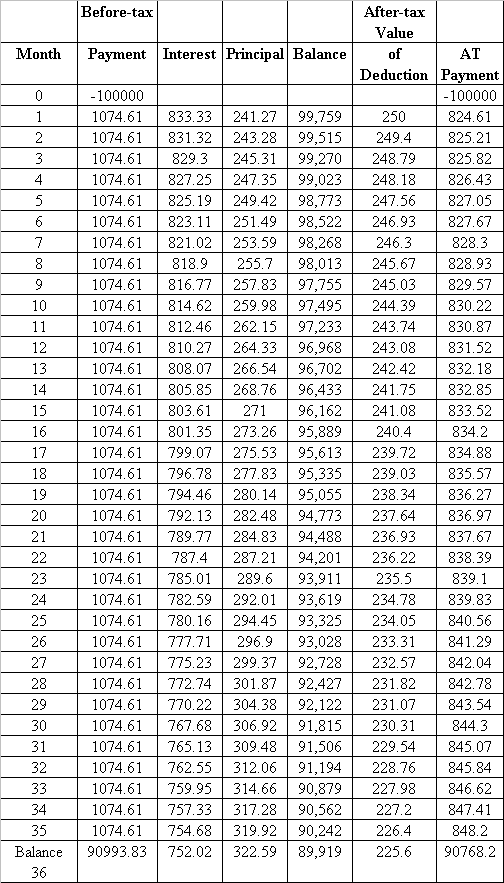The general formula to evaluate the after tax effective interest rate on loan is,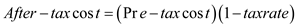With the substitution of given values in the above equation we get after tax interest rate as,b. By assuming the charge of 5 percent points which were deductible when they are paid, the after tax effective cost would be,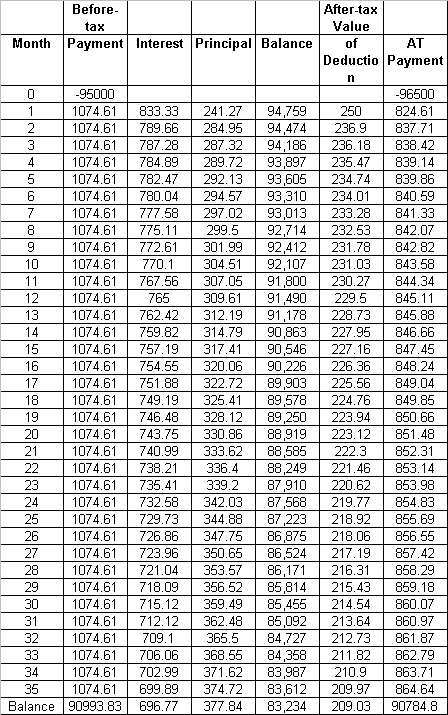Here in this case with the application of IRR rule for the values in after tax payment column, the effective cost after tax is found to be 8.56%. c. The before tax effective cost due to increase in 5 percentage points is not 12.09% and this also resulted in increased after tax effective cost with affect to 5 percentage points. The approximate after tax effective cost would be around,But however with the increase in 5 percentage points after tax effective cost is 0.0856. Thus the percentage of 5 points increase resulted in an increase of before and after tax effective costs.
Cost of fund borrowings arise when funds required are took by the borrower through mortgage. These costs get incremented when the borrower plans to procure additional funds through second mortgage. The cost on mortgage for a borrower includes interest along with the principal payment. The cost of borrowings gets incremented when the mortgages value as well as interest on mortgage increases. a. Here let us assume the purchase price of home be \$100,000. So the choice is between 80 percent loan of \$80,000 or 90 percent loan of \$90,000. Alternative I: 90 percent loan Given, Interest rate per annum - 8.5% which indicates monthly interest rate of 0.7083 % Loan term - 25 years or 300 months Loan amount - \$90,000 Calculation of monthly payments: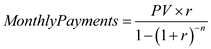Alternative II: 80 percent loan Given, Interest rate per annum - 8.5% which indicates monthly interest rate of 0.7083 % Loan term - 25 years or 300 months Loan amount - \$80,000 Calculation of monthly payments:Difference between loan amounts: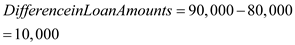Difference in Monthly Payments: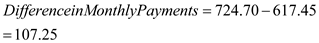Therefore the incremental cost of borrowing is 12.3% b. The following table shows the difference in monthly payments.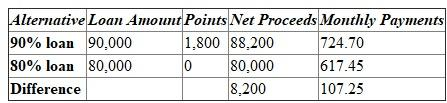Therefore the incremental cost of borrowing with charge of points would be 15.4% c. The following table shows the difference in monthly payments.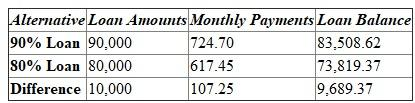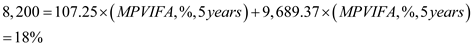Therefore the incremental cost after considering both increased points and change in tenure would be 18%. Note: The MPVIFA formula used helps out in finding the interest rate, using the present value of interest rate in annuity.